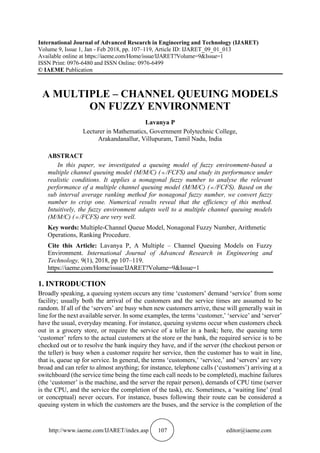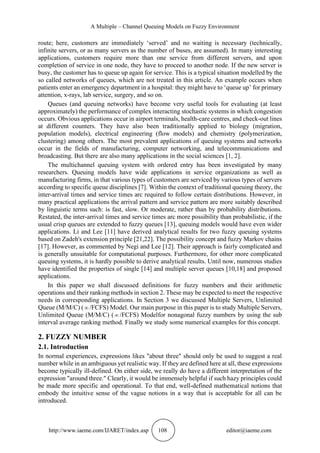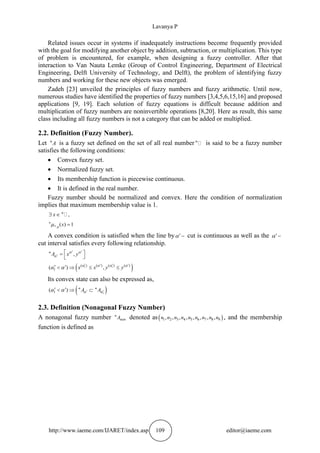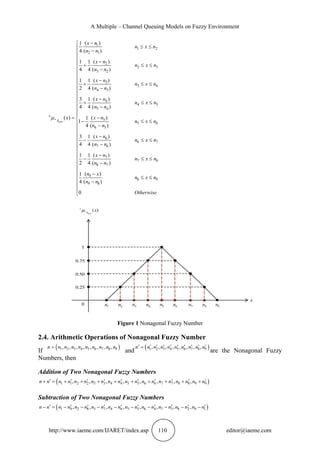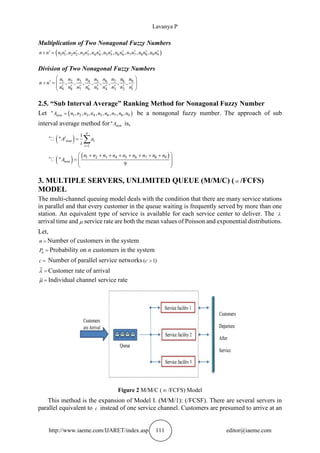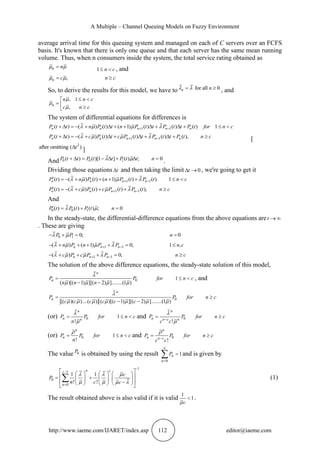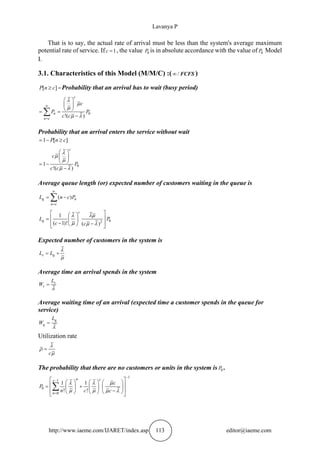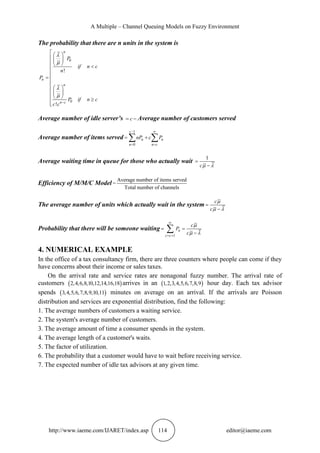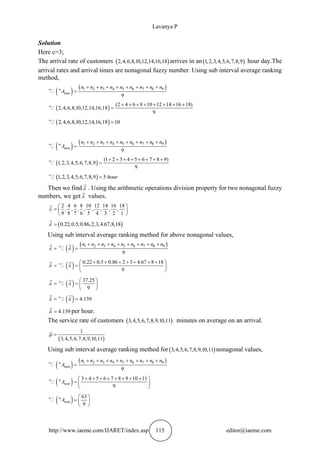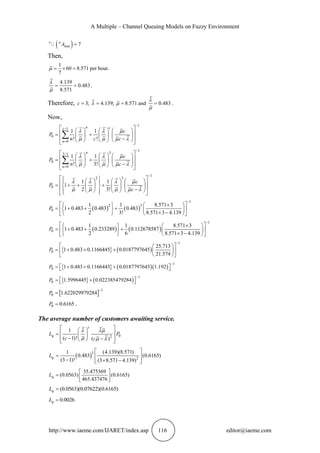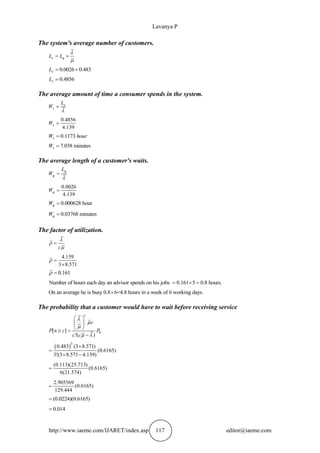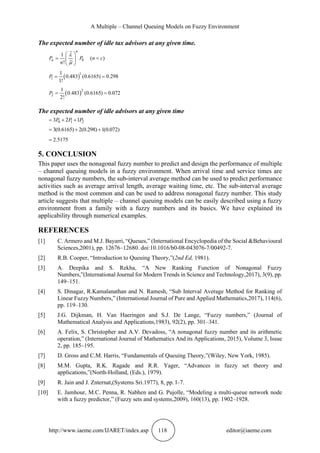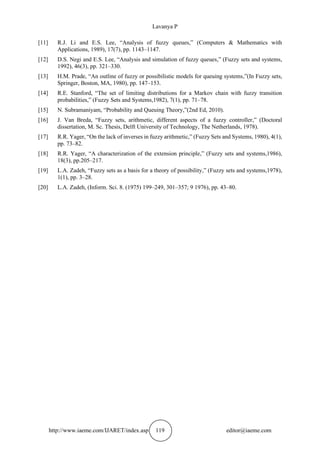Próximo SlideShare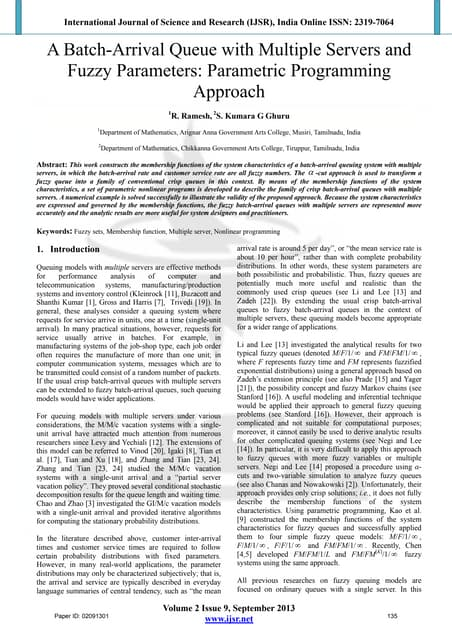A Batch-Arrival Queue with Multiple Servers and Fuzzy Parameters: Parametric ...
Cargando en ... 3
1 de 13

### A MULTIPLE – CHANNEL QUEUING MODELS ON FUZZY ENVIRONMENT

1. http://www.iaeme.com/IJARET/index.asp 107 editor@iaeme.com International Journal of Advanced Research in Engineering and Technology (IJARET) Volume 9, Issue 1, Jan - Feb 2018, pp. 107–119, Article ID: IJARET_09_01_013 Available online at https://iaeme.com/Home/issue/IJARET?Volume=9&Issue=1 ISSN Print: 0976-6480 and ISSN Online: 0976-6499 © IAEME Publication A MULTIPLE – CHANNEL QUEUING MODELS ON FUZZY ENVIRONMENT Lavanya P Lecturer in Mathematics, Government Polytechnic College, Arakandanallur, Villupuram, Tamil Nadu, India ABSTRACT In this paper, we investigated a queuing model of fuzzy environment-based a multiple channel queuing model (M/M/C) ( /FCFS) and study its performance under realistic conditions. It applies a nonagonal fuzzy number to analyse the relevant performance of a multiple channel queuing model (M/M/C) ( /FCFS). Based on the sub interval average ranking method for nonagonal fuzzy number, we convert fuzzy number to crisp one. Numerical results reveal that the efficiency of this method. Intuitively, the fuzzy environment adapts well to a multiple channel queuing models (M/M/C) ( /FCFS) are very well. Key words: Multiple-Channel Queue Model, Nonagonal Fuzzy Number, Arithmetic Operations, Ranking Procedure. Cite this Article: Lavanya P, A Multiple – Channel Queuing Models on Fuzzy Environment. International Journal of Advanced Research in Engineering and Technology, 9(1), 2018, pp 107–119. https://iaeme.com/Home/issue/IJARET?Volume=9&Issue=1 1. INTRODUCTION Broadly speaking, a queuing system occurs any time ‘customers’ demand ‘service’ from some facility; usually both the arrival of the customers and the service times are assumed to be random. If all of the ‘servers’ are busy when new customers arrive, these will generally wait in line for the next available server. In some examples, the terms ‘customer,’ ‘service’ and ‘server’ have the usual, everyday meaning. For instance, queuing systems occur when customers check out in a grocery store, or require the service of a teller in a bank; here, the queuing term ‘customer’ refers to the actual customers at the store or the bank, the required service is to be checked out or to resolve the bank inquiry they have, and if the server (the checkout person or the teller) is busy when a customer require her service, then the customer has to wait in line, that is, queue up for service. In general, the terms ‘customers,’ ‘service,’ and ‘servers’ are very broad and can refer to almost anything; for instance, telephone calls (‘customers’) arriving at a switchboard (the service time being the time each call needs to be completed), machine failures (the ‘customer’ is the machine, and the server the repair person), demands of CPU time (server is the CPU, and the service the completion of the task), etc. Sometimes, a ‘waiting line’ (real or conceptual) never occurs. For instance, buses following their route can be considered a queuing system in which the customers are the buses, and the service is the completion of the
2. A Multiple – Channel Queuing Models on Fuzzy Environment http://www.iaeme.com/IJARET/index.asp 108 editor@iaeme.com route; here, customers are immediately ‘served’ and no waiting is necessary (technically, infinite servers, or as many servers as the number of buses, are assumed). In many interesting applications, customers require more than one service from different servers, and upon completion of service in one node, they have to proceed to another node. If the new server is busy, the customer has to queue up again for service. This is a typical situation modelled by the so called networks of queues, which are not treated in this article. An example occurs when patients enter an emergency department in a hospital: they might have to ‘queue up’ for primary attention, x-rays, lab service, surgery, and so on. Queues (and queuing networks) have become very useful tools for evaluating (at least approximately) the performance of complex interacting stochastic systems in which congestion occurs. Obvious applications occur in airport terminals, health-care centres, and check-out lines at different counters. They have also been traditionally applied to biology (migration, population models), electrical engineering (flow models) and chemistry (polymerization, clustering) among others. The most prevalent applications of queuing systems and networks occur in the fields of manufacturing, computer networking, and telecommunications and broadcasting. But there are also many applications in the social sciences [1, 2]. The multichannel queuing system with ordered entry has been investigated by many researchers. Queuing models have wide applications in service organizations as well as manufacturing firms, in that various types of customers are serviced by various types of servers according to specific queue disciplines . Within the context of traditional queuing theory, the inter-arrival times and service times arc required to follow certain distributions. However, in many practical applications the arrival pattern and service pattern are more suitably described by linguistic terms such: is fast, slow. Or moderate, rather than by probability distributions. Restated, the inter-arrival times and service times arc more possibility than probabilistic, if the usual crisp queues are extended to fuzzy queues , queuing models would have even wider applications. Li and Lee  have derived analytical results for two fuzzy queuing systems based on Zadeh's extension principle [21,22]. The possibility concept and fuzzy Markov chains . However, as commented by Negi and Lee . Their approach is fairly complicated and is generally unsuitable for computational purposes. Furthermore, for other more complicated queuing systems, it is hardly possible to derive analytical results. Until now, numerous studies have identified the properties of single  and multiple server queues [10,18] and proposed applications. In this paper we shall discussed definitions for fuzzy numbers and their arithmetic operations and their ranking methods in section 2. These may be expected to meet the respective needs in corresponding applications. In Section 3 we discussed Multiple Servers, Unlimited Queue (M/M/C) ( /FCFS) Model. Our main purpose in this paper is to study Multiple Servers, Unlimited Queue (M/M/C) (  /FCFS) Modelfor nonagonal fuzzy numbers by using the sub interval average ranking method. Finally we study some numerical examples for this concept. 2. FUZZY NUMBER 2.1. Introduction In normal experiences, expressions likes "about three" should only be used to suggest a real number while in an ambiguous yet realistic way. If they are defined here at all, these expressions become typically ill-defined. On either side, we really do have a different interpretation of the expression "around three." Clearly, it would be immensely helpful if such hazy principles could be made more specific and operational. To that end, well-defined mathematical notions that embody the intuitive sense of the vague notions in a way that is acceptable for all can be introduced.
3. Lavanya P http://www.iaeme.com/IJARET/index.asp 109 editor@iaeme.com Related issues occur in systems if inadequately instructions become frequently provided with the goal for modifying another object by addition, subtraction, or multiplication. This type of problem is encountered, for example, when designing a fuzzy controller. After that interaction to Van Nauta Lemke (Group of Control Engineering, Department of Electrical Engineering, Delft University of Technology, and Delft), the problem of identifying fuzzy numbers and working for these new objects was emerged. Zadeh  unveiled the principles of fuzzy numbers and fuzzy arithmetic. Until now, numerous studies have identified the properties of fuzzy numbers [3,4,5,6,15,16] and proposed applications [9, 19]. Each solution of fuzzy equations is difficult because addition and multiplication of fuzzy numbers are noninvertible operations [8,20]. Here as result, this same class including all fuzzy numbers is not a category that can be added or multiplied. 2.2. Definition (Fuzzy Number). Let « A is a fuzzy set defined on the set of all real number « is said to be a fuzzy number satisfies the following conditions: • Convex fuzzy set. • Normalized fuzzy set. • Its membership function is piecewise continuous. • It is defined in the real number. Fuzzy number should be normalized and convex. Here the condition of normalization implies that maximum membership value is 1. ,   « x « ( ) 1 = « A x  A convex condition is satisfied when the line by −  cut is continuous as well as the −  cut interval satisfies every following relationship. ,      =   « A x y    ( ) 1 1 ( ) ( ) ( ) ( ) 1 ( ) ,           x x y y       Its convex state can also be expressed as, ) ( 1 1 ( )        « « A A     2.3. Definition (Nonagonal Fuzzy Number) A nonagonal fuzzy number « non A denoted as ) ( 1 2 3 4 5 6 7 8 9 , , , , , , , , n n n n n n n n n , and the membership function is defined as
4. A Multiple – Channel Queuing Models on Fuzzy Environment http://www.iaeme.com/IJARET/index.asp 110 editor@iaeme.com 1 1 2 2 1 2 2 3 3 2 3 3 4 4 3 4 4 5 5 4 « 5 5 6 6 5 6 6 7 7 6 7 7 8 8 7 9 8 9 8 ( ) 1 4 ( ) ( ) 1 1 4 4 ( ) ( ) 1 1 2 4 ( ) ( ) 3 1 4 4 ( ) ( ) ( ) 1 1 4 ( ) ( ) 3 1 4 4 ( ) ( ) 1 1 2 4 ( ) ( ) 1 4 ( ) −   − − +   − − +   − − +   − − = −   − − −   − − −   − −  − « non A x n n x n n n x n n x n n n x n n x n n n x n n x n n n x n x n x n n n x n n x n n n x n n x n n n n x n n n  9 0                                 x n Otherwise Figure 1 Nonagonal Fuzzy Number 2.4. Arithmetic Operations of Nonagonal Fuzzy Number If ) ( 1 2 3 4 5 6 7 8 9 , , , , , , , , = n n n n n n n n n n and ) ( 1 2 3 4 5 6 7 8 9 , , , , , , , ,           = n n n n n n n n n n are the Nonagonal Fuzzy Numbers, then Addition of Two Nonagonal Fuzzy Numbers ) ( 1 1 2 2 3 3 4 4 5 5 6 6 7 7 8 8 9 9 , , , , , , , ,           + = + + + + + + + + + n n n n n n n n n n n n n n n n n n n n Subtraction of Two Nonagonal Fuzzy Numbers ) ( 1 9 2 8 3 7 4 6 5 5 6 4 7 3 8 2 9 1 , , , , , , , ,           − = − − − − − − − − − n n n n n n n n n n n n n n n n n n n n
5. Lavanya P http://www.iaeme.com/IJARET/index.asp 111 editor@iaeme.com Multiplication of Two Nonagonal Fuzzy Numbers ) ( 1 1 2 2 3 3 4 4 5 5 6 6 7 7 8 8 9 9 , , , , , , , ,            = n n n n n n n n n n n n n n n n n n n n Division of Two Nonagonal Fuzzy Numbers 3 5 6 7 8 9 1 2 4 9 8 7 6 5 4 3 2 1 , , , , , , , ,     =               n n n n n n n n n n n n n n n n n n n n 2.5. “Sub Interval Average” Ranking Method for Nonagonal Fuzzy Number Let ) ( 1 2 3 4 5 6 7 8 9 , , , , , , , , = « non A n n n n n n n n n be a nonagonal fuzzy number. The approach of sub interval average method for « non A is, ( ) « 1 1 = =  « n i non i i A a i ( ) ( ) 1 2 3 4 5 6 7 8 9 « 9   + + + + + + + + =       « non n n n n n n n n n A 3. MULTIPLE SERVERS, UNLIMITED QUEUE (M/M/C) ( /FCFS) MODEL The multi-channel queuing model deals with the condition that there are many service stations in parallel and that every customer in the queue waiting is frequently served by more than one station. An equivalent type of service is available for each service center to deliver. The  arrival time and  service rate are both the mean values of Poisson and exponential distributions. Let, = n Number of customers in the system = n P Probability on n customers in the system = c Number of parallel service networks ( 1)  c =  Customer rate of arrival =  Individual channel service rate Figure 2 M/M/C (  /FCFS) Model This method is the expansion of Model I. (M/M/1): (/FCSF). There are several servers in parallel equivalent to c instead of one service channel. Customers are presumed to arrive at an
6. A Multiple – Channel Queuing Models on Fuzzy Environment http://www.iaeme.com/IJARET/index.asp 112 editor@iaeme.com average arrival time for this queuing system and managed on each of C servers over an FCFS basis. It's known that there is only one queue and that each server has the same mean running volume. Thus, when n consumers inside the system, the total service rating obtained as = n n   1  n c , and , =  n c n c   So, to derive the results for this model, we have to for all 0 =  n n   , and , 1 ,    =    n n n c c n c    The system of differential equations for differences is 1 1 ( ) ( ) ( ) ( 1) ( ) ( ) ( ) 1 + − +  = − +  + +  +  +   n n n n n P t t n P t t n P t t P t t P t for n c     1 1 ( ) ( ) ( ) ( ) ( ) ( ), + − +  = − +  +  +  +  n n n n n P t t c P t t c P t t P t t P t n c     [ 2 after omitting ( ) t ] And 0 0 1 ( ) ( )[1 ] ( ) ; 0 +  = −  +  = P t t P t t P t t n   . Dividing those equations t and then taking the limit 0  → t , we're going to get it 1 1 ( ) ( ) ( ) ( 1) ( ) ( ) 1 + −  = − + + + +   n n n n P t n P t n P t P t n c     1 1 ( ) ( ) ( ) ( ) ( ), + −  = − + + +  n n n n P t c P t c P t P t n c     And 0 0 1 ( ) ( ) ( ) ; 0  = + = P t P t P t n   In the steady-state, the differential-difference equations from the above equations are →  t . These are giving 0 1 0; 0 − + = = P P n   1 1 ( ) ( 1) 0, 1 , + − − + + + + =  n n n n P n P P n c     1 1 ( ) 0, + − − + + + =  n n n c P c P P n c     The solution of the above difference equations, the steady-state solution of this model, 0 1 ( )[( 1) ][( 2) ]........(1 ) =   − − n n P P for n c n n n      , and 0 [( )( )....( )] ( )[( 1) ][( 2) ]........(1 ) =  − − n n P P for n c c c c c c c         (or) 0 1 ! =   n n n P P for n c n   and 0 ! − =  n n n c n P P for n c c c   (or) 0 1 ! =   n n P P for n c n  and 0 ! − =  n n n c P P for n c c c  The value 0 P is obtained by using the result 0 1  = =  n n P and is given by 1 1 0 0 1 1 ! ! − − =           = +         −          n c c n c P n c c        (1) The result obtained above is also valid if it is valid 1 1  c  .
7. Lavanya P http://www.iaeme.com/IJARET/index.asp 113 editor@iaeme.com That is to say, the actual rate of arrival must be less than the system's average maximum potential rate of service. If 1 = c , the value 0 P is in absolute accordance with the value of 0 P Model I. 3.1. Characteristics of this Model (M/M/C) :( / FCFS  ) [ ]  = P n c Probability that an arrival has to wait (busy period) 0 !( )  =       = = −  c n n c c P P c c      Probability that an arrival enters the service without wait 1 [ ] = −  P n c 0 1 !( )       = − − c c P c c      Average queue length (or) expected number of customers waiting in the queue is ( )  = = −  q n n c L n c P 0 2 1 ( 1)! ( )       =   −   −     c q L P c c      Expected number of customers in the system is = + s q L L   Average time an arrival spends in the system = s s L W  Average waiting time of an arrival (expected time a customer spends in the queue for service) = q q L W  Utilization rate = c    The probability that there are no customers or units in the system is 0 P . 1 1 0 0 1 1 ! ! − − =           = +         −          n c c n c P n c c       
8. A Multiple – Channel Queuing Models on Fuzzy Environment http://www.iaeme.com/IJARET/index.asp 114 editor@iaeme.com The probability that there are n units in the system is 0 0 ! ! −             =               n n n n c P if n c n P P if n c c c     Average number of idle server’s = − c Average number of customers served Average number of items served 1 0 −  = = = +   c n n n n c nP c P Average waiting time in queue for those who actually wait 1 = − c  Efficiency of M/M/C Model Average number of items served = Total number of channels The average number of units which actually wait in the system = − c c    Probability that there will be someone waiting 1  = + = = −  n c c c P c    4. NUMERICAL EXAMPLE In the office of a tax consultancy firm, there are three counters where people can come if they have concerns about their income or sales taxes. On the arrival rate and service rates are nonagonal fuzzy number. The arrival rate of customers ( ) 2,4,6,8,10,12,14,16,18 arrives in an ( ) 1,2,3,4,5,6,7,8,9 hour day. Each tax advisor spends ( ) 3,4,5,6,7,8,9,10,11 minutes on average on an arrival. If the arrivals are Poisson distribution and services are exponential distribution, find the following: 1. The average numbers of customers a waiting service. 2. The system's average number of customers. 3. The average amount of time a consumer spends in the system. 4. The average length of a customer's waits. 5. The factor of utilization. 6. The probability that a customer would have to wait before receiving service. 7. The expected number of idle tax advisors at any given time.
9. Lavanya P http://www.iaeme.com/IJARET/index.asp 115 editor@iaeme.com Solution Here c=3; The arrival rate of customers ( ) 2,4,6,8,10,12,14,16,18 arrives in an( ) 1,2,3,4,5,6,7,8,9 hour day.The arrival rates and arrival times are nonagonal fuzzy number. Using sub interval average ranking method, ( ) ( ) 1 2 3 4 5 6 7 8 9 « 9 + + + + + + + + = « non n n n n n n n n n A ( ) « (2 4 6 8 10 12 14 16 18) 2,4,6,8,10,12,14,16,18 9 + + + + + + + + = ( ) « 2,4,6,8,10,12,14,16,18 10 = ( ) ( ) 1 2 3 4 5 6 7 8 9 « 9 + + + + + + + + = « non n n n n n n n n n A ( ) « (1 2 3 4 5 6 7 8 9) 1,2,3,4,5,6,7,8,9 9 + + + + + + + + = ( ) « 1,2,3,4,5,6,7,8,9 5 = hour Then we find  . Using the arithmetic operations division property for two nonagonal fuzzy numbers, we get  values. 2 4 6 8 10 12 14 16 18 , , , , , , , , 9 8 7 6 5 4 3 2 1   =      ( ) 0.22,0.5,0.86,2,3,4.67,8,18 =  Using sub interval average ranking method for above nonagonal values, ( ) ( ) 1 2 3 4 5 6 7 8 9 « 9 + + + + + + + + = = n n n n n n n n n   ( ) « 0.22 0.5 0.86 2 3 4.67 8 18 9 + + + + + + +   = =       ( ) « 37.25 9   = =       ( ) « 4.139 = =   4.139 =  per hour. The service rate of customers ( ) 3,4,5,6,7,8,9,10,11 minutes on average on an arrival. ( ) 1 3,4,5,6,7,8,9,10,11 =  Using sub interval average ranking method for( ) 3,4,5,6,7,8,9,10,11 nonagonal values, ( ) ( ) 1 2 3 4 5 6 7 8 9 « 9 + + + + + + + + = « non n n n n n n n n n A ( ) « 3 4 5 6 7 8 9 10 11 9 + + + + + + + +   =     « non A ( ) « 63 9   =     « non A
10. A Multiple – Channel Queuing Models on Fuzzy Environment http://www.iaeme.com/IJARET/index.asp 116 editor@iaeme.com ( ) « 7 = « nan A Then, 1 60 8.571 per hour. 7 =  =  4.139 0.483 8.571 = =   . Therefore, 3; 4.139; 8.571 and 0.483 = = = = c     . Now, 1 1 0 0 1 1 ! ! − − =           = +         −          n c c n c P n c c        1 3 3 1 0 0 1 1 ! 3! − − =           = +         −          n n c P n c        1 2 3 0 1 1 1 2 3! −               = + + +           −             c P c          ( ) ( ) 1 2 3 0 1 1 8.571 3 1 0.483 0.483 0.483 2 3! 8.571 3 4.139 −        = + + +        −       P ( ) ( ) 1 0 1 1 8.571 3 1 0.483 0.233289 0.112678587 2 6 8.571 3 4.139 −        = + + +        −       P   ( ) 1 0 25.713 1 0.483 0.1166445 0.0187797645 21.574 −     = + + +         P   ( )( ) 1 0 1 0.483 0.1166445 0.0187797645 1.192 −   = + + +   P   ( ) 1 0 1.5996445 0.022385479284 −   = +   P   1 0 1.622029979284 − = P 0 0.6165 = P . The average number of customers awaiting service. 0 2 1 ( 1)! ( )       =   −   −     c q L P c c      ( )3 2 1 (4.139)(8.571) 0.483 (0.6165) (3 1)! (3 8.571 4.139)   =   −  −     q L 35.475369 (0.0563) (0.6165) 465.437476   =     q L (0.0563)(0.07622)(0.6165) = q L 0.0026 = q L
11. Lavanya P http://www.iaeme.com/IJARET/index.asp 117 editor@iaeme.com The system's average number of customers. = + s q L L   0.0026 0.483 = + s L 0.4856 = s L The average amount of time a consumer spends in the system. = s s L W  0.4856 4.139 = s W 0.1173 = s W hour 7.038 minutes = s W The average length of a customer's waits. = q q L W  0.0026 4.139 = q W 0.000628 hour = q W 0.03768 minutes = q W The factor of utilization. = c    4.139 3 8.571 =   0.161 =  Number of hours each day an advisor spends on his jobs 0.161 5 0.8 hours. =  = On an average he is busy 0.8 6=4.8 hours in a week of 6 working days.  The probability that a customer would have to wait before receiving service 0 [ ] !( )        = − c c P n c P c c      ( )3 0.483 (3 8.571) (0.6165) 3!(3 8.571 4.139)  =  − (0.113)(25.713) (0.6165) 6(21.574) = 2.905569 (0.6165) 129.444 = (0.0224)(0.6165) = 0.014 =
12. A Multiple – Channel Queuing Models on Fuzzy Environment http://www.iaeme.com/IJARET/index.asp 118 editor@iaeme.com The expected number of idle tax advisors at any given time. 0 1 ( ) !   =      n n P P n c n   ( )1 1 1 0.483 (0.6165) 0.298 1! = = P ( )2 2 1 0.483 (0.6165) 0.072 2! = = P The expected number of idle advisors at any given time 0 1 2 3 2 1 = + + P P P 3(0.6165) 2(0.298) 1(0.072) = + + 2.5175 = 5. CONCLUSION This paper uses the nonagonal fuzzy number to predict and design the performance of multiple – channel queuing models in a fuzzy environment. When arrival time and service times are nonagonal fuzzy numbers, the sub-interval average method can be used to predict performance activities such as average arrival length, average waiting time, etc. The sub-interval average method is the most common and can be used to address nonagonal fuzzy number. This study article suggests that multiple – channel queuing models can be easily described using a fuzzy environment from a family with a fuzzy numbers and its basics. We have explained its applicability through numerical examples. REFERENCES  C. Armero and M.J. Bayarri, “Queues,” (International Encyclopedia of the Social &Behavioural Sciences,2001), pp. 12676–12680. doi:10.1016/b0-08-043076-7/00492-7.  R.B. Cooper, “Introduction to Queuing Theory,”(2nd Ed, 1981).  A. Deepika and S. Rekha, “A New Ranking Function of Nonagonal Fuzzy Numbers,”(International Journal for Modern Trends in Science and Technology,2017), 3(9), pp. 149–151.  S. Dinagar, R.Kamalanathan and N. Ramesh, “Sub Interval Average Method for Ranking of Linear Fuzzy Numbers,” (International Journal of Pure and Applied Mathematics,2017), 114(6), pp. 119–130.  J.G. Dijkman, H. Van Haeringen and S.J. De Lange, “Fuzzy numbers,” (Journal of Mathematical Analysis and Applications,1983), 92(2), pp. 301–341.  A. Felix, S. Christopher and A.V. Devadoss, “A nonagonal fuzzy number and its arithmetic operation,” (International Journal of Mathematics And its Applications, 2015), Volume 3, Issue 2, pp. 185–195.  D. Gross and C.M. Harris, “Fundamentals of Queuing Theory,”(Wiley, New York, 1985).  M.M. Gupta, R.K. Ragade and R.R. Yager, “Advances in fuzzy set theory and applications,”(North-Holland, (Eds.), 1979).  R. Jain and J. Znternat,(Systems Sri.1977), 8, pp. l–7.  E. Jamhour, M.C. Penna, R. Nabhen and G. Pujolle, “Modeling a multi-queue network node with a fuzzy predictor,” (Fuzzy sets and systems,2009), 160(13), pp. 1902–1928.
13. Lavanya P http://www.iaeme.com/IJARET/index.asp 119 editor@iaeme.com  R.J. Li and E.S. Lee, “Analysis of fuzzy queues,” (Computers & Mathematics with Applications, 1989), 17(7), pp. 1143–1147.  D.S. Negi and E.S. Lee, “Analysis and simulation of fuzzy queues,” (Fuzzy sets and systems, 1992), 46(3), pp. 321–330.  H.M. Prade, “An outline of fuzzy or possibilistic models for queuing systems,”(In Fuzzy sets, Springer, Boston, MA, 1980), pp. 147–153.  R.E. Stanford, “The set of limiting distributions for a Markov chain with fuzzy transition probabilities,” (Fuzzy Sets and Systems,1982), 7(1), pp. 71–78.  N. Subramaniyam, “Probability and Queuing Theory,”(2nd Ed, 2010).  J. Van Breda, “Fuzzy sets, arithmetic, different aspects of a fuzzy controller,” (Doctoral dissertation, M. Sc. Thesis, Delft University of Technology, The Netherlands, 1978).  R.R. Yager, “On the lack of inverses in fuzzy arithmetic,” (Fuzzy Sets and Systems, 1980), 4(1), pp. 73–82.  R.R. Yager, “A characterization of the extension principle,” (Fuzzy sets and systems,1986), 18(3), pp.205–217.  L.A. Zadeh, “Fuzzy sets as a basis for a theory of possibility,” (Fuzzy sets and systems,1978), 1(1), pp. 3–28.  L.A. Zadeh, (Inform. Sci. 8. (1975) 199–249, 301–357; 9 1976), pp. 43–80.